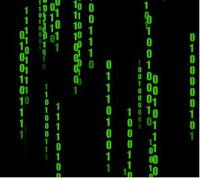# 进制转换–详解## 一、正数

### 1. 十 —–> 二

（25.625）（十）

25/2=12……1
12/2=6 ……0
6/2=3 ……0
3/2=1 ……1
1/2=0 ……1

0.625*2=1.25
0.25 *2=0.5
0.5 *2=1.0

(1) 10/2,商5余0；
(2) 5/2,商2余1；
(3)2/2,商1余0；
(4)1／2，商0余1．
(5)将所得的余数值倒过来，就是1010，所以十进制的10转化为二进制就是1010 .

### 2. 二 —-> 十

（11001.101）（二）

1*2（4）+1*2（3）+0*2（2）+0*2（1）+1*2（0）=25

1*2（-1）+0*2（-2）+1*2（-3）=0.625

(1) 1乘以2的0次方，等于1；
(2) 1乘以2的2次方，等于4；
(3) 1乘以2的4次方，等于16；
(4) 1乘以2的5次方，等于32；
(5) 将这些结果相加：1＋4＋16＋32＝53.

（25.625）（十）

25/8=3……1
3/8 =0……3

0.625*8=5

（31.5）（八）

3*8（1）+1*8（0）=25

5*[8（-1）]=0.625

### 5. 十 —-> 十六

（25.625）（十）

25/16=1……9
1/16 =0……1

0.625*16=10（即十六进制的A或a）

### 6. 十六—-> 十

（19.A）（十六）

1*16（1）+9*16（0）=25

10*16（-1）=0.625       （10代表A）

ASCII码：A：65，0：48，以此类推。

（11001.101）（二）

001=1
011=3

101=5

（31.5）（八）

1—->1—->001
3—->11

5—->101

（19.A）（十六）

9—->1001
1—->0001（相当于1）

A(即10)—->1010

（11001.101）（二）

1001—->9
0001—->1

1010—->10—->A

## 二、负数

-9的补码为11110111。然后三位一划
111—->7
110—->6
011—->3

0.8*16=12.8
0.8*16=12.8
.
.
.
.
.

N=(dmdm-1…d1d0)-R
=dm*(-R)^m+dm-1*(-R)^m-1+…+d1*(-R)^1+d0*(-R)^0
15=1*(-2)^4+0*(-2)^3+0*(-2)^2+1*(-2)^1+1*(-2)^0
=10011(-2)

## C程序代码：（支持负进制）

#include <stdio.h>
#include <math.h>
main()
{
long n,m,r;
while( scanf( “%ld%ld”,&n,&r)!=EOF){
if (abs(r)> 1 && !(n <0 && r> 0)){
long result;
long *p=result;
printf( “%ld=”,n);
if (n!=0){
while(n!=0){
m=n/r;*p=n-m*r;
if (*p <0 && r <0){
*p=*p+abs(r);m++;
}
p++;n=m;
}
for (m=p-result-1;m>=0;m–){
if (result[m]> 9)
printf( “%c”,55+result[m]);
else
printf( “%d”,result[m]);
}
}
else printf( “0”);
printf( “(base%d)n”,r);
} }
return 0;
}

#include<stdio.h>
int x;
int jzzh(int y,int ml)
{
int i,j;
i=ml;
int a;
x=0;
for(a=1;;a++)
{
j=i%y;
if(i!=0)
{
x[a]=j;
x++;
}
else
break;
i=i/y;
}
}
int main()
{
long int y,ml;
long int a;
printf(“请输入需要转换至进制数：”);
scanf(“%d”,&y);
printf(“请输入数字：”);
scanf(“%d”,&ml);
jzzh(y,ml);
for(a=x;a>=1;a–)
printf(“%d”,x[a]);
printf(“n”);
system(“pause”);
}